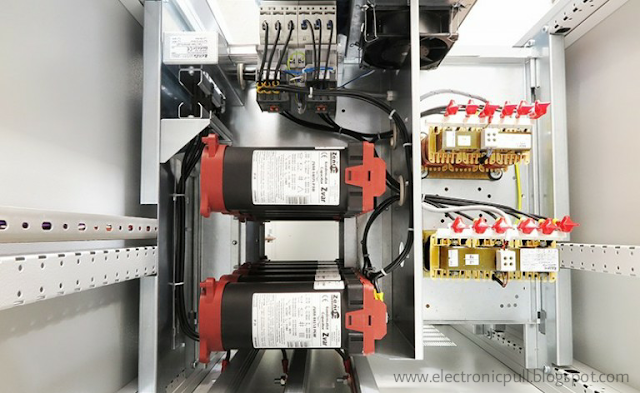#Why Do We Care So Much About Power Factor Correction? (on photo: The capacitor battery with the case open; credit: zenex.pl)

## Power factor improvement:

Power factor, as every electrical engineer should know, is defined as the ratio of real power to volt-amperes and is the cosine of the phase angle between the voltage and current in an AC circuit. These are neatly defined quantities with sinusoidal voltages and currents. Power factor can be improved by adding capacitors on the power line to draw a leading current and supply lagging vars to the system.
Power factor correction capacitors can be switched in and out as necessary to maintain var and voltage control.
Many utilities charge industrial customers a certain rate for kilowatt-hours of energy consumed in a month, and another charge related to the infrastructure necessary to supply that power under the customer’s conditions of operation.
If the customer is operating with a low power factor load, the demand charge is higher, because the current requirement is higher. Table 1 below shows the differences between energy and demand.
Table 1 – Energy and Demand
 Energy Demand Kilowatt-hours from: Kilovoltamperes from: – Tons of coal Generators – Acre-feed of water Transmission lines – Barrels of oil Transformers – Cubic feet of gas Switchgear – Ounces of uranium Distribution systems

Power factor penalties on demand charges range from none to a factor of 2 on the peak power demand.
Figure 1 shows a power factor demand penalty imposed by one utility. The factor is F= 0.8 + 0.6 kvarh/kWh, but not less than 1 and not more than 2. Typical values for power charges are \$0.03 to \$0.05 cents per kilowatt-hour and \$5.00 to \$15.00 per month per kilowatt-hour of power factor corrected demand.

Figure 1 – Demand multiplier (Credit : EEP)
The pain of a poor power factor can be shown by an example. If a plant utilizes 100,000 kW-h in a month, the energy charge at\$0.04 per kW-h is \$4000. If it has a peak demand of 400 kW during the month, the demand charge at unity power factor with a \$10.00/kW rate is also \$4000. If the power factor is only 0.70, the demand bill goes up to \$5650, a 41% increase.
Power factor correction is usually a paying proposition.
Figure 2 shows a demand of 400 kW at a power factor of 0.70 as above. The kVA demand is 571 kVA, and the var demand is 408 kvar.Figure 2 – Power factor correction (Credit :EEP)

If a 300 kvar capacitor bank is added to the supply, the net kvar demand goes down to108 kvar, demand is now 415 kVA, the power factor is now 0.965and there is no power factor penalty.
Another benefit of power factor correction is that feeder voltage regulation is improved. Adding capacitors will reduce the quadrature currents, those currents that are 90° out of phase with the line voltage, but overcorrection can cause problems.
Many utilities are glad to get capacitors on distribution feeders, especially if they are switched on only during working hours. But some have problems with excessive voltage rise during periods of light load, and some may have tariffs that discriminate against leading power factors, too.
The same kVA capacity is required for a given leading load power factor as for a lagging one.
Some plant engineers have a pathological fear of a leading power factor for reasons the author could never fathom. In point of fact, no load has any idea what the power factor is and couldn’t care less.
It has been scientifically proven that leading power factors do not cause illness to machines or humans. The only possible concern for a leading power factor is overvoltage at light load if the power factor goes too far leading.
In a large plant, power factor correction can be applied at the interface between the utility and the customer, the point of common coupling, as it is termed. This is generally less expensive than adding a lot of small capacitor banks, particularly when switchgear is required or harmonics are present.
Reference // Power Electronics Design: A Practitioner’s Guide by Keith H. Sueker